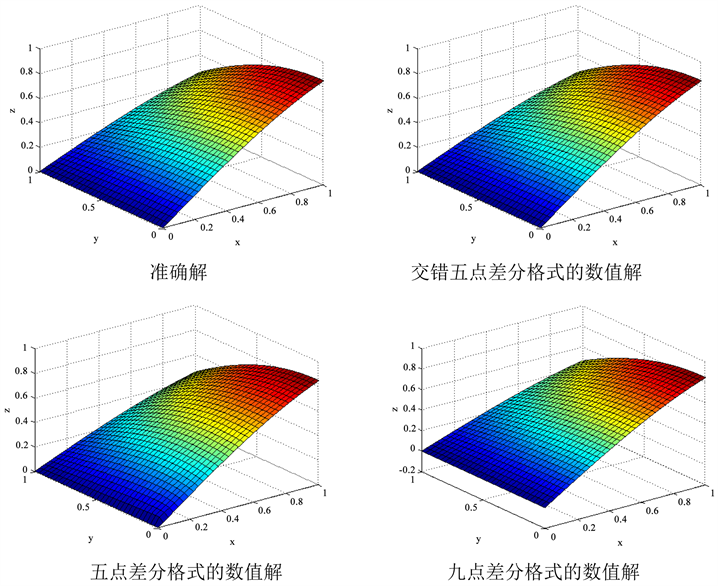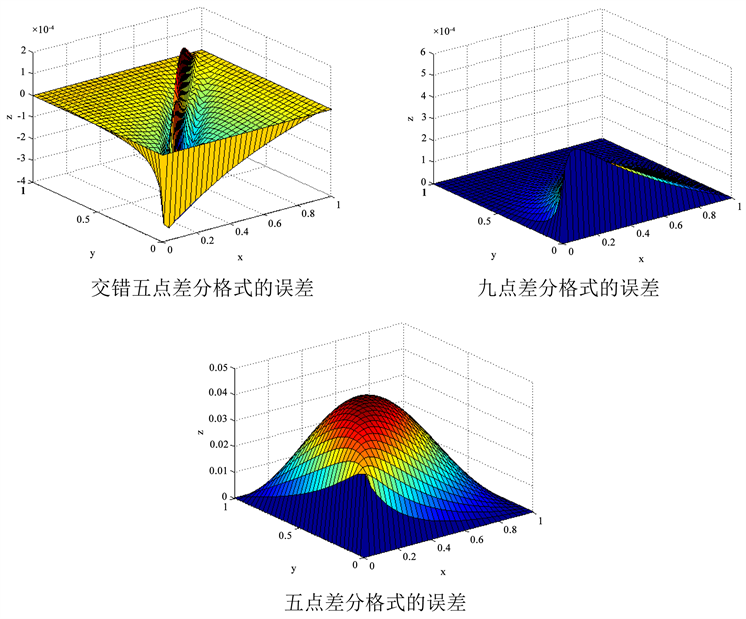﻿ 变系数椭圆型方程定解问题的一种数值解法 A Numerical Solution to a Solution Problem for Elliptic Equation with Variable Coefficients

Vol. 07  No. 10 ( 2018 ), Article ID: 27253 , 9 pages
10.12677/AAM.2018.710151

A Numerical Solution to a Solution Problem for Elliptic Equation with Variable Coefficients

Duowei Zhu1, Adil Nazakat1, Mamat Imam2, Abduwali Abdirixit1

1College of Mathematics and Systems Science, Xinjiang University, Urumqi Xinjiang

2College of Mathematics and Information, Shaanxi Normal University, Xi’an ShaanxiReceived: Oct. 1st, 2018; accepted: Oct. 17th, 2018; published: Oct. 24th, 2018ABSTRACT

This paper proposes a numerical solution for solving that problem of fixed solution of the variable coefficient elliptic equation, and we get the corresponding error analysis, the method is verified by numerical experiments; convergence speed and small error, in time and space can achieve second-order accuracy.

Keywords:Variable Coefficient Elliptic Equation, Numerical Solution, The Error Analysis

1新疆大学，数学与系统科学学院，新疆 乌鲁木齐

2陕西师范大学，数学与信息学院，陕西 西安1. 引言

$\left\{\begin{array}{l}-\underset{i=1}{\overset{2}{\sum }}\underset{j=1}{\overset{2}{\sum }}\frac{\partial }{\partial {x}_{i}}\left({a}_{ij}\left({x}_{1},{x}_{2}\right)\frac{\partial u\left({x}_{1},{x}_{2}\right)}{\partial {x}_{j}}\right)=f\left({x}_{i},{x}_{j}\right),\forall \left({x}_{1},{x}_{2}\right)\in \Omega ,\\ {u\left({x}_{1},{x}_{2}\right)|}_{\Gamma }=g\left({x}_{1},{x}_{2}\right),\forall \left({x}_{1},{x}_{2}\right)\in \Gamma .\end{array}$ (1)

2. 差分格式的建立

${x}_{1i}=ih,i=0,1,2,\cdots ,m,$

${x}_{2j}=jk,j=0,1,2,\cdots n,$

${\Gamma }_{h}$ 表示网格线 ${x}_{1}={x}_{1i}$${x}_{2}={x}_{2j}$$\Gamma$ 的交点的集合，并称此类点为边界节点。

${\Omega }_{h}$ 上的网格函数为：

$U=\left\{{U}_{ij}|0\le i\le m,0\le j\le n\right\}$

${U}_{ij}=\left\{u\left({x}_{1i},{x}_{2j}\right)|0\le i\le m,0\le j\le n\right\}.$

2.1. 交错五点差分格式

${\left[\frac{1}{r}\frac{\partial }{\partial r}\left(r\frac{\partial u}{\partial r}\right)\right]}_{ij}\approx \frac{1}{{r}_{i}{\left(\Delta r\right)}^{2}}\left[{r}_{i+\frac{1}{2}}u\left({r}_{i+1,{\theta }_{j}}\right)-\left({r}_{i+\frac{1}{2}}+{r}_{i-\frac{1}{2}}\right)u\left({r}_{i,{\theta }_{j}}\right)+{r}_{i-\frac{1}{2}}u\left({r}_{i-1,{\theta }_{j}}\right)\right]$ (2)

$\frac{\left({a}_{ij}\left({x}_{1},{x}_{2}\right)\frac{\partial u\left({x}_{1},{x}_{2}\right)}{\partial {x}_{j}}\right)|{}_{\left(i+\frac{1}{2},j\right)}-\left({a}_{ij}\left({x}_{1},{x}_{2}\right)\frac{\partial u\left({x}_{1},{x}_{2}\right)}{\partial {x}_{j}}\right)|{}_{\left(i-\frac{1}{2},j\right)}}{h},$

$\frac{\partial }{\partial {x}_{1}}\left({a}_{11}\frac{\partial u}{\partial {x}_{1}}\right)=\frac{{a}_{i+\frac{1}{2},j}^{11}\left({u}_{i+1,j}-{u}_{i,j}\right)-{a}_{i-\frac{1}{2},j}^{11}\left({u}_{i,j}-{u}_{i-1,j}\right)}{{h}^{2}}+O\left({h}^{2}\right),$ (3)

$\frac{\partial }{\partial {x}_{1}}\left({a}_{12}\frac{\partial u}{\partial {x}_{2}}\right)=\frac{{a}_{i+\frac{1}{2},j}^{12}\left({u}_{i+\frac{1}{2},j+\frac{1}{2}}-{u}_{i+\frac{1}{2},j-\frac{1}{2}}\right)-{a}_{i-\frac{1}{2},j}^{12}\left({u}_{i-\frac{1}{2},j+\frac{1}{2}}-{u}_{i-\frac{1}{2},j-\frac{1}{2}}\right)}{{h}^{2}}+O\left(kh\right),$ (4)

$\frac{\partial }{\partial x{}_{2}}\left({a}_{21}\frac{\partial u}{\partial {x}_{1}}\right)=\frac{{a}_{i,j+\frac{1}{2}}^{21}\left({u}_{i+\frac{1}{2},j+\frac{1}{2}}-{u}_{i-\frac{1}{2},j+\frac{1}{2}}\right)-{a}_{i,j-\frac{1}{2}}^{21}\left({u}_{i+\frac{1}{2},j-\frac{1}{2}}-{u}_{i-\frac{1}{2},j-\frac{1}{2}}\right)}{{h}^{2}}+O\left(kh\right),$ (5)

$\frac{\partial }{\partial {x}_{2}}\left({a}_{22}\frac{\partial u}{\partial {x}_{2}}\right)=\frac{{a}_{i,j+\frac{1}{2}}^{22}\left({u}_{i,j+1}-{u}_{i,j}\right)-{a}_{i,j-\frac{1}{2}}^{22}\left({u}_{i,j}-{u}_{i,j-1}\right)}{{k}^{2}}+O\left({k}^{2}\right),$ (6)

${a}_{i+\frac{1}{2},j}^{11},{a}_{i+\frac{1}{2},j}^{12},{a}_{i,j+\frac{1}{2}}^{21},{a}_{i,j+\frac{1}{2}}^{22},{a}_{i-\frac{1}{2},j}^{11},{a}_{i-\frac{1}{2},j}^{12},{a}_{i,j-\frac{1}{2}}^{21},{a}_{i,j-\frac{1}{2}}^{22}.$

$\begin{array}{l}-\frac{{a}_{i+\frac{1}{2},j}^{11}}{{h}^{2}}{u}_{i+1,j}+\left(\frac{{a}_{i+\frac{1}{2},j}^{11}+{a}_{i-\frac{1}{2},j}^{11}}{{h}^{2}}+\frac{{a}_{i,j+\frac{1}{2}}^{22}+{a}_{i,j-\frac{1}{2}}^{22}}{{k}^{2}}\right){u}_{i,j}-\frac{{a}_{i-\frac{1}{2},j}^{11}}{{h}^{2}}{u}_{i-1,j}-\frac{{a}_{i,j+\frac{1}{2}}^{22}}{{k}^{2}}{u}_{i,j+1}\\ -\frac{{a}_{i,j-\frac{1}{2}}^{22}}{{k}^{2}}{u}_{i,j-1}-\left(\frac{{a}_{i+\frac{1}{2},j}^{12}+{a}_{i,j+\frac{1}{2}}^{21}}{kh}\right){u}_{i+\frac{1}{2},j+\frac{1}{2}}+\left(\frac{{a}_{i+\frac{1}{2},j}^{12}+{a}_{i,j-\frac{1}{2}}^{21}}{kh}\right){u}_{i+\frac{1}{2},j-\frac{1}{2}}\\ +\left(\frac{{a}_{i-\frac{1}{2},j}^{12}+{a}_{i,j+\frac{1}{2}}^{21}}{kh}\right){u}_{i-\frac{1}{2},j+\frac{1}{2}}-\left(\frac{{a}_{i-\frac{1}{2},j}^{12}+{a}_{i,j-\frac{1}{2}}^{21}}{kh}\right){u}_{i-\frac{1}{2},j-\frac{1}{2}}={f}_{i,j}\end{array}$ (7)

$\left(\left(i-\frac{1}{2},j-\frac{1}{2}\right),\left(i-\frac{1}{2},j+\frac{1}{2}\right),\left(i+\frac{1}{2},j-\frac{1}{2}\right),\left(i+\frac{1}{2},j+\frac{1}{2}\right)\right)$

(称为网格的中心点)交错得到的，从节点的角度看，用了五个节点 ${x}_{i,j+1},{x}_{i,j-1},{x}_{i,j},{x}_{i-1,j},{x}_{i+1,j}$ ，但是计算的时候我们用到了网格的中心点，又可以看作是九个点的差分格式。因此，我们称差分格式(7)为交错五点差分格式。

2.2. 五点差分格式

${u}_{i+\frac{1}{2},j+\frac{1}{2}}=\frac{{u}_{i+1,j}+{u}_{i,j+1}}{2}+O\left({h}^{2}+{k}^{2}\right),$ (8)

${u}_{i+\frac{1}{2},j-\frac{1}{2}}=\frac{{u}_{i+1,j}+{u}_{i,j-1}}{2}+O\left({h}^{2}+{k}^{2}\right),$ (9)

${u}_{i-\frac{1}{2},j+\frac{1}{2}}=\frac{{u}_{i-1,j}+{u}_{i,j+1}}{2}+O\left({h}^{2}+{k}^{2}\right),$ (10)

${u}_{i-\frac{1}{2},j-\frac{1}{2}}=\frac{{u}_{i-1,j}+{u}_{i,j-1}}{2}+O\left({h}^{2}+{k}^{2}\right),$ (11)

$\begin{array}{l}\left(-\frac{{a}_{i+\frac{1}{2},j}^{11}}{{h}^{2}}+\frac{{a}_{i,j-\frac{1}{2}}^{21}-{a}_{i,j+\frac{1}{2}}^{21}}{2kh}\right){u}_{i+1,j}+\left(\frac{{a}_{i+\frac{1}{2},j}^{11}+{a}_{i-\frac{1}{2},j}^{11}}{{h}^{2}}+\frac{{a}_{i,j+\frac{1}{2}}^{22}+{a}_{i,j-\frac{1}{2}}^{22}}{{k}^{2}}\right){u}_{i,j}+\left(-\frac{{a}_{i-\frac{1}{2},j}^{11}}{{h}^{2}}+\frac{{a}_{i,j+\frac{1}{2}}^{21}-{a}_{i,j-\frac{1}{2}}^{21}}{2kh}\right){u}_{i-1,j}\\ +\left(-\frac{{a}_{i,j+\frac{1}{2}}^{22}}{{k}^{2}}+\frac{{a}_{i-\frac{1}{2},j}^{12}-{a}_{i+\frac{1}{2},j}^{12}}{2kh}\right){u}_{i,j+1}+\left(-\frac{{a}_{i,j-\frac{1}{2}}^{22}}{{k}^{2}}+\frac{{a}_{i+\frac{1}{2},j}^{12}-{a}_{i-\frac{1}{2},j}^{12}}{2kh}\right){u}_{i,j-1}={f}_{i,j}\end{array}$ (12)

2.3. 九点差分格式

$\frac{\partial }{\partial {x}_{1}}\left({a}_{11}\frac{\partial u}{\partial {x}_{1}}\right)=\frac{{a}_{i+\frac{1}{2},j}^{11}\left({u}_{i+1,j}-{u}_{i,j}\right)-{a}_{i-\frac{1}{2},j}^{11}\left({u}_{i,j}-{u}_{i-1,j}\right)}{{h}^{2}}+O\left({h}^{2}\right),$ (13)

$\frac{\partial }{\partial {x}_{1}}\left({a}_{12}\frac{\partial u}{\partial {x}_{2}}\right)=\frac{{a}_{i+1,j}^{12}\left({u}_{i+1,j+1}-{u}_{i+1,j-1}\right)-{a}_{i-1,j}^{12}\left({u}_{i-1,j+1}-{u}_{i-1,j-1}\right)}{4kh}+O\left(kh\right),$ (14)

$\frac{\partial }{\partial {x}_{2}}\left({a}_{21}\frac{\partial u}{\partial {x}_{1}}\right)=\frac{{a}_{i,j+1}^{21}\left({u}_{i+1,j+1}-{u}_{i-1,j+1}\right)-{a}_{i,j-1}^{21}\left({u}_{i+1,j-1}-{u}_{i-1,j-1}\right)}{4kh}+O\left(kh\right),$ (15)

$\frac{\partial }{\partial {x}_{2}}\left({a}_{22}\frac{\partial u}{\partial {x}_{2}}\right)=\frac{{a}_{i,j+\frac{1}{2}}^{22}\left({u}_{i,j+1}-{u}_{i,j}\right)-{a}_{i,j-\frac{1}{2}}^{22}\left({u}_{i,j}-{u}_{i,j-1}\right)}{{h}^{2}}+O\left({k}^{2}\right),$ (16)

$\begin{array}{l}-\frac{{a}_{i+\frac{1}{2},j}^{11}}{{h}^{2}}{u}_{i+1,j}+\left(\frac{{a}_{i+\frac{1}{2},j}^{11}+{a}_{i-\frac{1}{2},j}^{11}}{{h}^{2}}+\frac{{a}_{i,j+\frac{1}{2}}^{22}+{a}_{i,j-\frac{1}{2}}^{22}}{{k}^{2}}\right){u}_{i,j}-\frac{{a}_{i-\frac{1}{2},j}^{11}}{{h}^{2}}{u}_{i-1,j}-\frac{{a}_{i,j-\frac{1}{2}}^{22}}{{k}^{2}}{u}_{i,j-1}\\ -\frac{{a}_{i,j+\frac{1}{2}}^{22}}{{k}^{2}}{u}_{i,j+1}-\left(\frac{{a}_{i+1,j}^{12}+{a}_{i,j+1}^{21}}{4kh}\right){u}_{i+1,j+1}+\left(\frac{{a}_{i+1,j}^{12}+{a}_{i,j-1}^{21}}{4kh}\right){u}_{i+1,j-1}\\ +\left(\frac{{a}_{i-1,j}^{12}+{a}_{i,j+1}^{21}}{4kh}\right){u}_{i-1,j+1}-\left(\frac{{a}_{i-1,j}^{12}+{a}_{i,j-1}^{21}}{4kh}\right){u}_{i-1,j-1}={f}_{i,j}\end{array}$ (17)

3. 差分格式的误差分析

$\begin{array}{c}T\left({x}_{1},{x}_{2}\right)=-\frac{{h}^{2}}{6}\frac{\partial {a}_{11}}{\partial {x}_{1}}\frac{{\partial }^{3}u}{\partial {x}_{1}{}^{3}}\left(\xi ,{x}_{2}\right)-\frac{{k}^{2}}{6}\frac{\partial {a}_{22}}{\partial {x}_{2}}\frac{{\partial }^{3}u}{\partial {x}_{2}{}^{3}}\left({x}_{1},\eta \right)-\frac{{h}^{2}}{24}\frac{\partial {a}_{21}}{\partial {x}_{2}}\frac{{\partial }^{3}u}{\partial {x}_{1}{}^{3}}\left(\xi ,{x}_{2}\right)\\ \text{\hspace{0.17em}}\text{\hspace{0.17em}}-\frac{{k}^{2}}{24}\frac{\partial {a}_{12}}{\partial {x}_{1}}\frac{{\partial }^{3}u}{\partial {x}_{2}{}^{3}}\left({x}_{1},\eta \right)-\frac{{h}^{2}}{8}\frac{\partial {a}_{12}}{\partial {x}_{1}}\frac{{\partial }^{2}u}{\partial {x}_{1}{}^{2}}\left(\xi ,{x}_{2}\right)\frac{\partial u}{\partial {x}_{2}}\left({x}_{1},\eta \right)\\ \text{\hspace{0.17em}}\text{\hspace{0.17em}}-\frac{{k}^{2}}{8}\frac{\partial {a}_{21}}{\partial {x}_{2}}\frac{{\partial }^{2}u}{\partial {x}_{2}{}^{2}}\left({x}_{1},\eta \right)\frac{\partial u}{\partial {x}_{1}}\left(\xi ,{x}_{2}\right)\end{array}$ (18)

$T\left({x}_{1},{x}_{2}\right)=-\frac{{h}^{2}}{6}\left(\frac{\partial {a}_{11}}{\partial {x}_{1}}+\frac{\partial {a}_{21}}{\partial {x}_{2}}\right)\frac{{\partial }^{3}u}{\partial {x}_{1}{}^{3}}\left(\xi ,{x}_{2}\right)-\frac{{k}^{2}}{6}\left(\frac{\partial {a}_{22}}{\partial {x}_{2}}+\frac{\partial {a}_{12}}{\partial {x}_{1}}\right)\frac{{\partial }^{3}u}{\partial {x}_{2}{}^{3}}\left({x}_{1},\eta \right)$ (19)

$\begin{array}{c}T\left({x}_{1},{x}_{2}\right)=-\frac{{h}^{2}}{6}\frac{\partial {a}_{11}}{\partial {x}_{1}}\frac{{\partial }^{3}u}{\partial {x}_{1}{}^{3}}\left(\xi ,{x}_{2}\right)-\frac{{k}^{2}}{6}\frac{\partial {a}_{22}}{\partial {x}_{2}}\frac{{\partial }^{3}u}{\partial {x}_{2}{}^{3}}\left({x}_{1},\eta \right)-\frac{{h}^{2}}{12}\frac{\partial {a}_{21}}{\partial {x}_{2}}\frac{{\partial }^{3}u}{\partial {x}_{1}{}^{3}}\left(\xi ,{x}_{2}\right)\\ \text{\hspace{0.17em}}\text{\hspace{0.17em}}-\frac{{k}^{2}}{12}\frac{\partial {a}_{12}}{\partial {x}_{1}}\frac{{\partial }^{3}u}{\partial {x}_{2}{}^{3}}\left({x}_{1},\eta \right)-\frac{{h}^{2}}{12}\frac{\partial {a}_{12}}{\partial {x}_{1}}\frac{{\partial }^{2}u}{\partial {x}_{1}{}^{2}}\left(\xi ,{x}_{2}\right)\frac{\partial u}{\partial {x}_{2}}\left({x}_{1},\eta \right)\\ \text{\hspace{0.17em}}\text{\hspace{0.17em}}-\frac{{k}^{2}}{12}\frac{\partial {a}_{21}}{\partial {x}_{2}}\frac{{\partial }^{2}u}{\partial {x}_{2}{}^{2}}\left({x}_{1},\eta \right)\frac{\partial u}{\partial {x}_{1}}\left(\xi ,{x}_{2}\right)\end{array}$ (20)

4. 数值例子

$\left\{\begin{array}{l}-\underset{i=1}{\overset{2}{\sum }}\underset{j=1}{\overset{2}{\sum }}\frac{\partial }{\partial {x}_{1}}\left({a}_{i,j}\left({x}_{1},{x}_{2}\right)\frac{\partial u\left({x}_{1},{x}_{2}\right)}{\partial {x}_{j}}\right)=f\left({x}_{1},{x}_{2}\right),\forall \left({x}_{1},{x}_{2}\right)\in \Omega ,\\ {a}_{i,j}\left({x}_{1},{x}_{2}\right)={x}_{i}{x}_{j},i,j=1,2,\\ f\left({x}_{1},{x}_{2}\right)=-3{x}_{1}\mathrm{cos}{x}_{1}\mathrm{cos}{x}_{2}+{x}_{1}^{2}\mathrm{sin}{x}_{1}\mathrm{cos}{x}_{2}+3{x}_{2}\mathrm{sin}{x}_{1}\mathrm{sin}{x}_{2}+2{x}_{1}{x}_{2}\mathrm{cos}{x}_{1}\mathrm{sin}{x}_{2}+{x}_{2}^{2}\mathrm{sin}{x}_{1}\mathrm{cos}{x}_{2},\\ {u\left({x}_{1},{x}_{2}\right)|}_{\Gamma }=0,\left({x}_{1},{x}_{2}\right)\in \partial \Omega \end{array}$ (21)Table 1. Exact solution and numerical solution of three difference schemes

$M=N=32$ ，即 $h=k=\frac{1}{32}$ ，用上述的三种差分格式解问题(1)，得到的准确解与数值解的图像如图1

$M=N=32$ ，即 $h=k=\frac{1}{32}$ ，用上述的三种差分格式求解问题(1)，得到准确解与数值解的误差如图2Figure 1. M = N = 32, Exact solution and numerical solution of three difference schemesTable 2. The number of iterations calculated by Gauss-Seidel and SOR iterative methods in three difference schemesTable 3. Error and error order of three difference schemesFigure 2. Error of three difference schemes

5. 结论

A Numerical Solution to a Solution Problem for Elliptic Equation with Variable Coefficients[J]. 应用数学进展, 2018, 07(10): 1299-1307. https://doi.org/10.12677/AAM.2018.710151

1. 1. 苏铭德. 用半直接法数值求解一类椭圆型方程[J]. 高等学校计算数学学报, 1984(3): 26-35.

2. 2. 何跃. 一类退化椭圆型方程边值问题的适定性[J]. 数学年刊A辑, 2007, 28(5): 651-666.

3. 3. 韩丕功. 关于半线性椭圆型方程和方程组的研究[J]. 中国科学院研究生院学报, 2009, 26(1): 141-143.

4. 4. Douglas, J. (1962) Alternating Discriminant Methods for Three Space Variable. Numerische Mathematik, 4, 41-63. https://doi.org/10.1007/BF01386295

5. 5. Peaceman, D.W. and Rachford, H.H. (1955) The Numerical Solution of Parabolic and Elliptic Difference Equation. Journal of the Society for Industrial and Applied Mathematics, 3, 28-41.

6. 6. Hou, S. and Liu, X.D. (2005) A Numerical Method for Solving Variable Coefficient Elliptic Equation with Interfaces. Journal of Computational Physics, 202, 411-445. https://doi.org/10.1016/j.jcp.2004.07.016

7. 7. 孙岩刚. 变系数椭圆型方程的紧差分格式[D]: [硕士学位论文]. 沈阳: 东北大学, 2008.

8. 8. Mohamed, N.A., Mohamed, N.F, Mohamed, N.H., et al. (2016) Numerical Solution of Dirichlet Boundary-Domain Integro-Differential Equation with Less Number of Collocation Points. Applied Mathematical Sciences, 10, 2459-2469. https://doi.org/10.12988/ams.2016.6381

9. 9. Raeli, A., Bergmann, M. and Iollo, A. (2017) A Finite-Difference Method for the Variable Coefficient Poisson Equation on Hierarchical Cartesian Meshes. Journal of Computational Physics, 355, 59-77. https://doi.org/10.1016/j.jcp.2017.11.007

10. 10. Gupta, H.S. (2012) A Numerical Study of Variable Coefficient Elliptic Cauchy Problem via Projection Method. International Journal of Computer Mathematics, 89, 795-809. https://doi.org/10.1080/00207160.2012.659426

11. 11. Ang, W.T., Kusuma, J. and Clements, D.L. (1995) A BIE for a Second Order Elliptic Partial Differential Equation with Variable Coefficients. Computational Mechanics. Springer Berlin Heidelberg, 2683-2688.